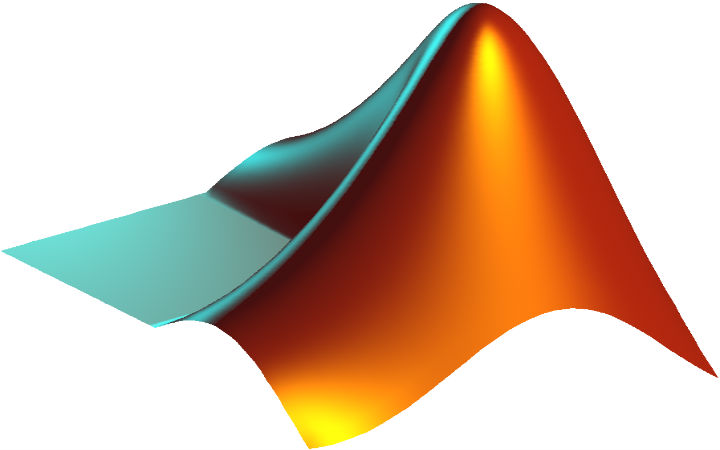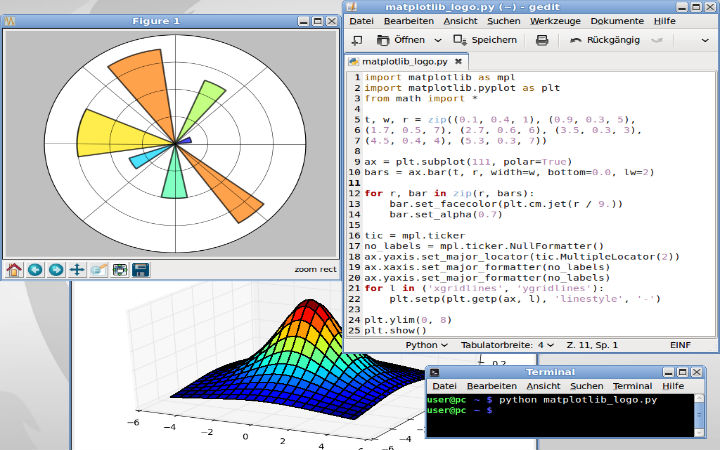# Matlab Simulation Projects for Research Scholars.

Matlab supports technical computing. It is easily accessible to visualization, programming and computing purposes. Wireless communication, medical imaging, signal processing, digital image processing and wireless sensor networks are the sub domains that comes under Matlab Simulation Projects. Projects on Matlab are offered by us to both under graduate and post graduate students and also research scholars. Results are produces as images, texts or graphs in Matlab Simulation Projects.

## Functions of Matlab Simulation Projects.

Various simulation processes are done with the help of matlab built in functions. They are as follows:

• Median: calculates median of the variable.
• Max: find Maximum value of data.
• Sum: Sum all content variables.
• Mode: calculates mode of the variable.
• Prod: multiplies the content.
• Std: calculates standard deviation of the variables.
• Size: provide size of the variable.
• Sqrt: calculating square root of the variable.
• Mean-Calculate mean of the variable### Matrices in Matlab Simulation:

A certain array of order should be followed while using matrix in matlab. The number 1 is used to number rows and columns. Specific data is held by floats or integer format. Any matrix can be assigned to any variable.

Matlab Commands for Managing a Session:

• Help – Searches for help topics.
• Whos – list down current variables for long display.
• Global – variables are declared in globally.
• Who – list current variables.
• Clear – eliminate variables from memory.
• Look for – searches help entries for a keyword.
• Ck – clear command window.
• Quit – matlab shall be stopped.
• Exist – checks for existence of file.

#### Filter and Thresholding.

Filter: Attributes from inputs are separated by filtering. Only these filtered images are further taken into account. High pass filter and low pass filter are the two types of filter. In image processing noises are smoothened and features are enhanced using filters.

Thresholding: It is the chief process of image segmentation. Optimal thresholding and iterative thresholding are few of thresholding methods. Histogram approximation is performed using optimal thresholding. The threshold value should always be in minimum.

Matlab Plotting: Generally a plot is among two vectors. The first vector is called as abscissa vector and the later one is said to be an ordinate vector. These vectors must have similar length.

##### Uses of Matlab Simulation Projects.
• Graphics in 2D and 3D which includes color and animation.
• Numerical solution of different equations.
• Modeling.
• Simulation.
• Prototyping.
• Forecast and Computations.

Flow of breast tumor detection.

• Collect mammogram image database from online.
• Pre-processing – Non-linear wiener filter.
• Pectoral Muscle Identification – MLO-view.
• Region Segmentation – Seeded Region Growing.
• Feature Extraction – GLCM.
• Feature Selection – Adaptive Neuro Fuzzy inference System (NAFIS).
• Tumor Classification – Benign and malignant.
###### Creating Matlab Simulation Projects?

Matlab Simulation projects are created based on default matlab functions, methods, procedures and mathematical calculations. Image processing, biomedical engineering, audio processing, video processing and geosciences remote sensing concepts are implemented using matlab simulation.

Medical imaging modalities are gathered from imaging centre, and it is in the format of 2D or 3D. Matlab is a high level programming language which has uses several specific toolboxes to perform image processing concepts in an easiest way.

0+
Total completed Matlab simulation Projects
0+
2014-15.Completed Matlab simulation Projects
0+
2015-16.Ongoing Matlab simulation Projects
Friendly atmosphere in the project center which made us easy to interact with the staffs and complete our project as per our requirement
Care for every student was given.I am happy with the project they delivered . Thanks a lot.
They guided us with technical concepts and also guided how to present a project Would recommend to others.
My project was delivered to me on time.I am extremely satisfied with the output .